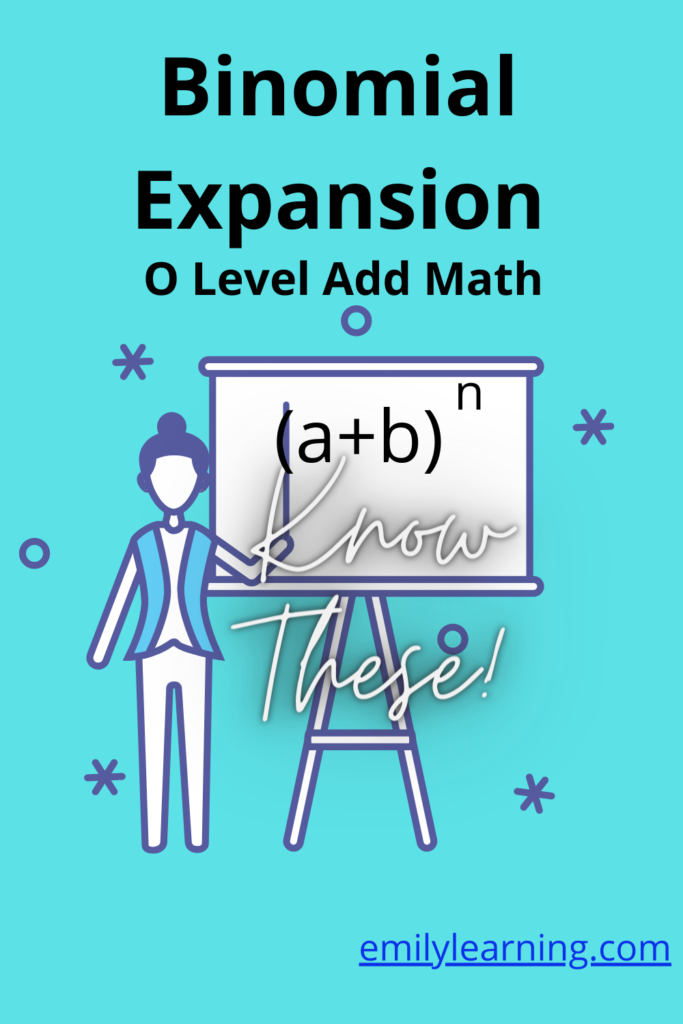# What you need to know for binomial expansion tested in O Level Additional MathematicsFor binomial expansion for O Level Additional Mathematics, you are tested only on binomial expansions with powers that are positive integers. Meaning if you have an expansion of this form (a+b)n, n is a positive integer for your syllabus. This is because the formula that you learn to apply for binomial is only applicable if n is a positive integer.

It’s a totally different expansion if we have negative powers, powers that are negative, or a combination of both. This (negative powers, or non-integer powers) is tested in A Level Mathematics.

There’s nothing to memorize for this chapter. Everything you need to know is in your formula booklet. What’s important is to understand how to apply the formula to the common questions.

In this chapter on binomial, you are tested on:

• binomial expansion and finding an expression of a certain term in the expansion
• knowledge of nCr and how to use it.

## Want to learn O Level Additional Math on-demand?

Check out our on-demand O Level Additional Math course here.

error: Content is protected !!